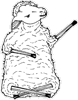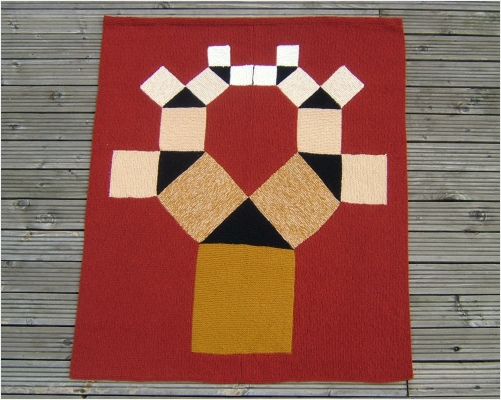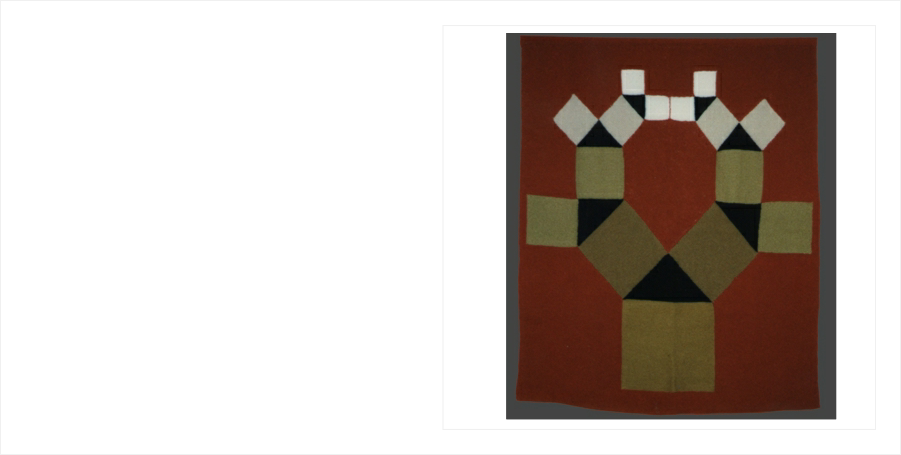﻿ Pythagoras TreeBuy nowOther places to visit

Order Form

Mental Blocks

The World of Illusion Knittingfor this afghan

on the order formBuy nowPYTHAGORAS TREE

Pythagoras Tree represents Pythagoras’ Theorem in the form of a fractal ‘tree’ design.

‘The square on the hypotenuse is equal to the sum of the squares on the other two sides.’

The original, which was bought by the Mathematics Collection of the London Science Museum, can be seen below.KNITTING INFORMATION

The first version (below) was knitted in Aran yarn; the second (above) in DK, using two strands together, to produce a toning range of colours.

The pattern includes ideas for more complex versions of the tree.Pythagoras TreeRELATED DESIGNS

PYTHAGORAS AFGHAN

Pythagorean Ripples

PYTHAGORAS CUSHION

The Other Two Sides

PUPPETS

Mr P & Mr FCONSTRUCTION INFORMATION

The ‘tree’ is knitted in one piece by picking up stitches from existing shapes. Only one yarn is used at a time.

The surround is knitted on afterwardsPythagoras’ Theorem states that the area of the squares on the two shorter sides of a right-angled triangle add up to the area of the square on the hypotenuse (longest side) of the triangle.

The black shapes in the design are right-angled triangles. They are also isosceles triangles because they have two equal sides. The two smaller squares are therefore the same size.

The design repeats itself with the small squares becoming the larger squares for the next part of the tree. This is similar to the way a fractal goes on repeating with smaller and smaller copies of itself. It is not a true fractal. Some parts of the fractal have been missed off to make the design fit on the afghan and to make it more closely resemble a tree.

In knitting terms, each small square has exactly half as many stitches to knit as the large square on the same triangle. There are five sizes of square, getting ever smaller.